### Symmetry-Solutions Ex-13.3

CBSE Class –VI Mathematics
NCERT Solutions
Chaper 13 Symmetry (Ex. 13.3)

Question 1. Find the number of lines of symmetry in each of the following shapes. How will you check your answer?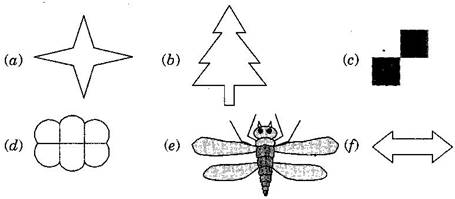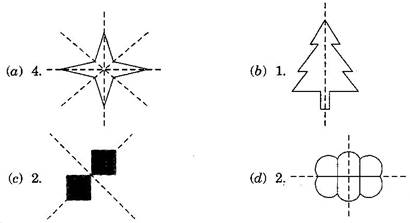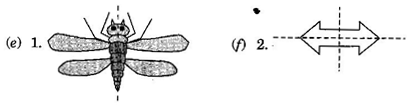The answers can be checked either by folding at the line of symmetry or by using a mirror on the mirror line.
Question 2. Copy the following drawing on squared paper. Complete each one of them such that the resulting figure has two dotted lines as two lines of symmetry.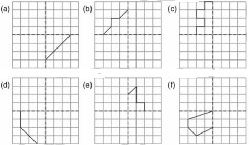How did you go about completing the picture?
Answer: Using the given lines of symmetry, we go about completing the picture.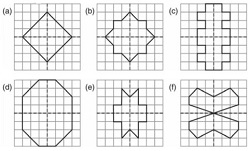Question 3.In each figure below, a letter of the English alphabet is shown along with a vertical line. Take the mirror image of the letter in the given line. Find which letters look the same after reflection (i.e., which letters look the same in the image) and which do not. Can you guess why?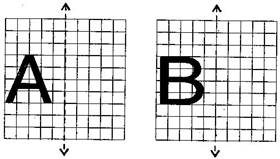Try for O E M N P H L T S V X
Answer: The mirror image of letters A and B in given line will look as shown below.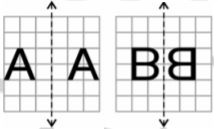We can see that after reflection A looks same but B does not look same. It is because of the letter A has a vertical line of symmetry where as in B there is no vertical line of symmetry.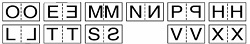it is clear that O, M, H, T, V and X look same after reflection, but E, P, L, and S do not. It is due to the letters E, P, L and S have no vertical line of symmetry. The letters which look same as reflection have a vertical line of symmetry.